# Vectors: Using Dot and Cross Product

## Problem

You’d like to understand what is meant by dot product and cross product.

## Solution

In this recipe we’ll introduce the concept of vector dot product and cross product and how they might be used.

### Dot product

Dot product is an operation on two vectors that returns a scalar. It is often visualized as the projection of vector A onto vector B: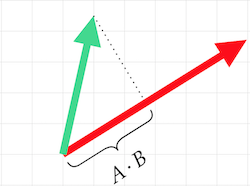This is the formula for calculating the dot product: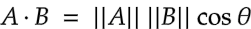Where `θ` is the angle between the two vectors and `||A||` is the magnitude of `A`.

This is very useful when both vectors are normalized (i.e. their magnitudes are `1`), then the formula simplifies to: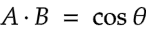This shows that the dot product is directly related to the angle between the two vectors. Since `cos(0) == 1` and `cos(180) == -1`, the result of the dot product can tell you how closely aligned two vectors are: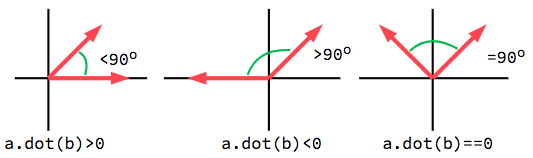See below for how we can apply this fact in a practical example.

### Cross product

The cross product of two vectors is a third vector that is perpendicular to both of them. Its magnitude is related to their magnitudes and the angle between them.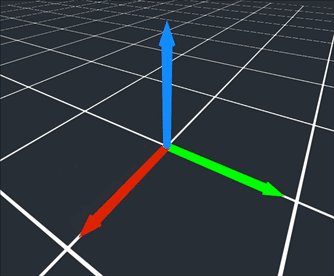Once again, if we’re using normalized vectors, the result is simplified: it will be directly related to the angle and its magnitude will range from -1 to 1.

### Practical applications

Consider this animation, showing how the results of `Vector2.dot()` and `Vector2.cross()` change in relation to the changing angle: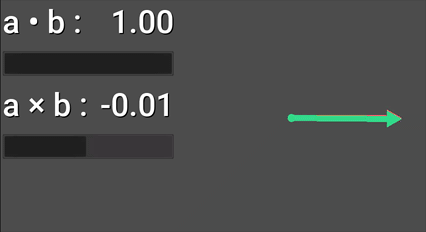This demonstrates two common applications of these methods. If the red vector is our object’s forward direction, and the green shows the direction towards another object:

• Dot product: Using the result, we can tell if the object is in front of (`> 0`) or behind (`< 0`) us.
• Cross product: Using the result, we can tell if the object is to the left (`> 0`) or right (`< 0`).